## Thursday, December 13, 2012

### Cluster levels of Categorical variable to avoid over-fitting

Consider this context: target variable target_revenue is a continuous variable. The predictors include continuous variables like hist_visits, as well as categorical variable like best_leafnode_id, which has hundreds of levels.

If use this best_leafnode_id directly, we may fit the data well because of the many levels of predictor best_leafnode_id(since there are lots of levels, and there is an estimation for each level, so it's like we have more parameters, and we have more degree of freedom, and thus the fitting will be better). As is shown in the second graph below.

Because we input too many parameters, one potential problem is over-fitting. That is, we fit the training data well but it will not predict well on the validation dataset.

From the second graph, if look at the training data, the reg7(reg with all levels of original data) has less MSE=83.95 which is less than reg6(regression with clustered levels) MSE=86.07. However, if we look at the validation data, we can see reg6(MSE=82.129) is less than reg7(MSE=105.655). That means if using the original data without clustering their levels, it will cause overfitting. It shows the clustered level method can avoid over-fitting. But we should choose a proper number of new levels. Not too small, not too large.

Below is the SAS code to cluster the huge level categorical variable: first calculate the mean of target_revenue at each level of best_leafnode_id, then bucket the levels of best_leafnode_id into less levels by the value of mean in the first step. Then we can format the old levels into new levels, the number of new levels is assigned by us.

Pic01 -- SAS code to cluster levelsPic02 -- Comparing the two models:

## Sunday, December 9, 2012

### R: Replicate plot with ggplot2 (part 4) bar chart

The original plot from UCLA ATS is here. Here the same plot is done in package ggplot2.

The bar chart here is to show how many observations for each level of ses(=1, 2 and 3 separately, will be replaced by "low", "median" and "high" separately).

The first plot is the plain bar chart:The second one is to replace the original value of ses by "low", "median" and "high" separately: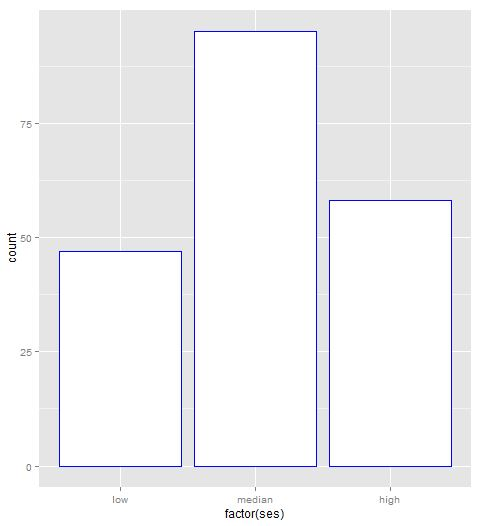The third one is to change the width of the bin.The forth is to group the data by female first, and then bin on each level of female(0 or 1). This is stacked plot. From the plot it is not easy to figure out which is higher. So we need to plot it separately as is shown in the fifth graph.The fifth one is plot bar chart at each level of female. That is, plot two bar chart for ses at 0 and 1 level of females separately.### R: Replicate plot with ggplot2 (part 3) boxplot

This is to replicate the boxplot from UCLA ATS:　Examples of box plots

The first is to draw the boxplot with no featuresThe second is filling the box area with blue color.The third is the box plot splited by a categorical variable ses: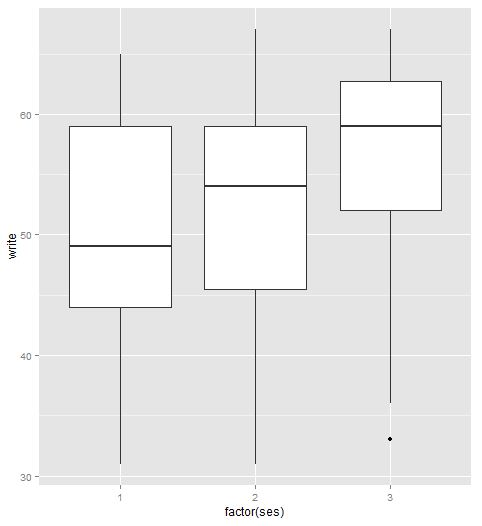Next is to rename the levels of the categorical variable: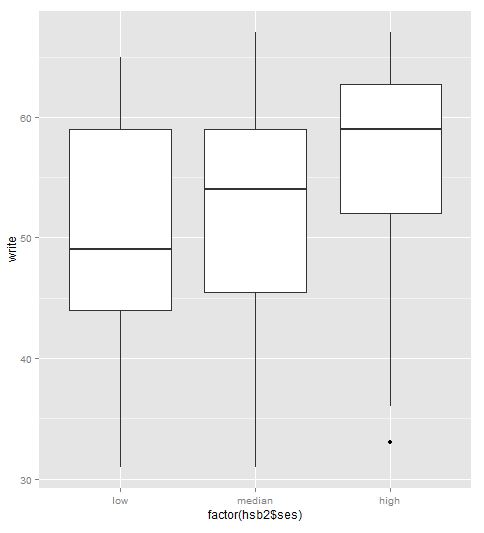The fifth one is to set notch = TRUEThe sixth is the plot on the interaction levels of more than one categorical variable:A little more, if we want to add outliers on the plot, it should be like:

## Saturday, December 8, 2012

### R: Replicate plot with ggplot2 (part 2) histogram, emprtical curve, normal density curve

The first one is to replicate the scatter plot here. This part is to replicat the plot of histogram.

The original UCLA ATS like is: http://www.ats.ucla.edu/stat/r/gbe/histogram.htm.

In ggplot2, geom_histogram is used to draw histogram.

The data is:

The first one is histogram with black fill:

The output graph is:ggplot2 has more choice that you can fill in the color by the counts in each bin. like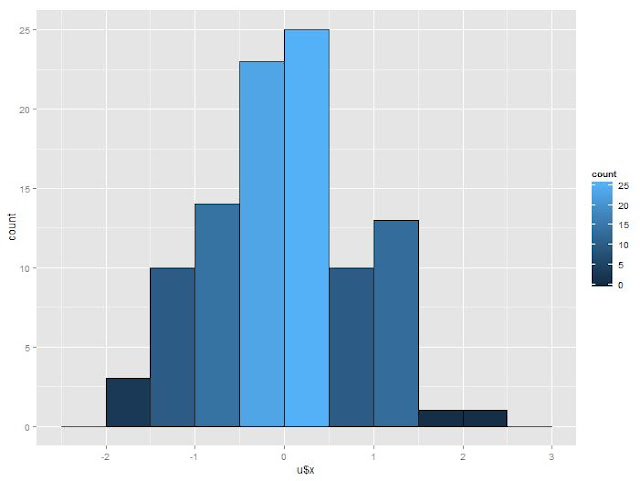Next is to change the binwidth. In ggplot2 this can be done by binwidth or by breaks. Here is shown by binwidth:Next is to change from frequency to density(percentage in fact). This can be done by assigning y to be ..density.. in ggplot2:Then is to add normal density and empirical curve in the plot.

First is the empirical curve: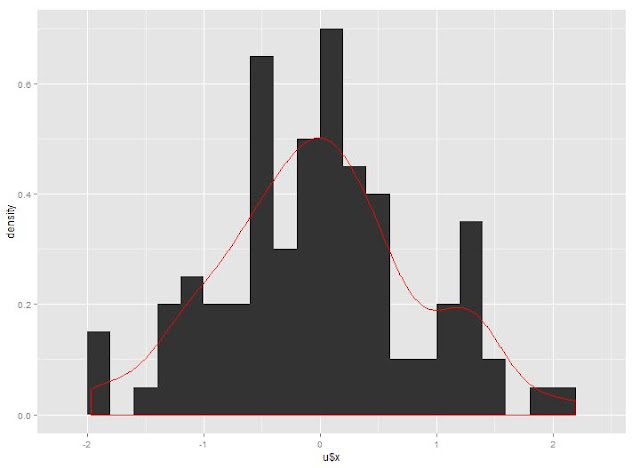Next is the Normal density with mean and std are from the generated data:The last one is to add the counts on top of each bin. I did not figure out how to do it. It should be easy by adding text. But unitl now my process has not reached there.

## Thursday, December 6, 2012

### R: check variables with missing data and how many missing(NA) data in a dataframe

It's easy to check with apply function:
```cat("\014")
rm(list=ls())